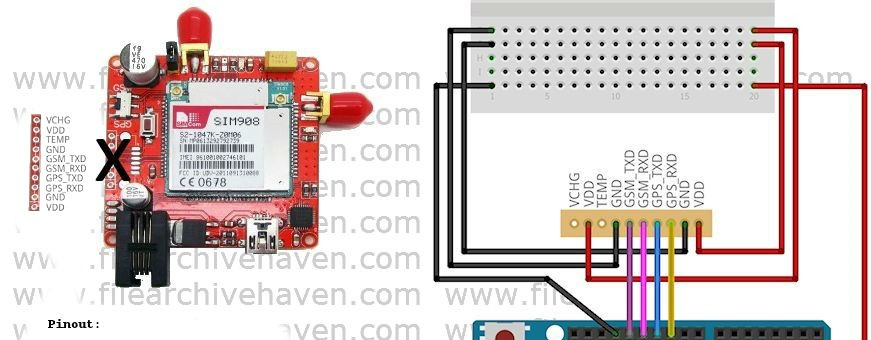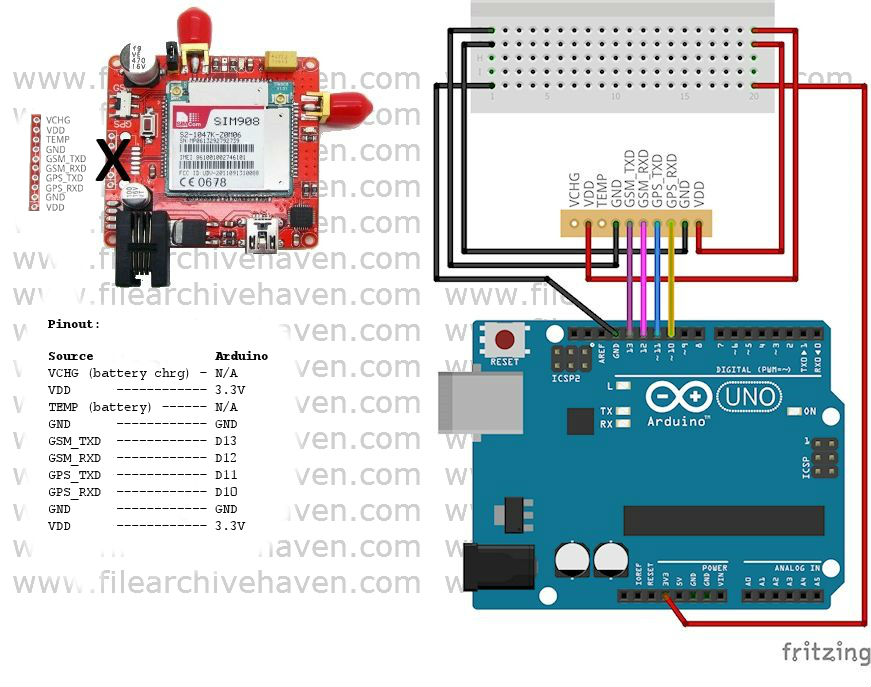# Working with GPS and GSM on Arduino–Part 1 of 2 (SIM908)Part 1:  Getting the GSM working

One of the projects I’m undertaking is a remote GPS tracker for my vehicle.

True, you can purchase these in the 30 dollar range from Deal Extreme if you search vehicle trackers.  However, I did see questions regarding temperature and stability.  And one of the worries for me is the websites used for tracking are individually controlled by small companies in China (which could go out of business at any moment).

With that said, GPS and GSM combo units from popular sites such as SparkFun, AdaFruit or CanadianRobotics are likely to run in the 100\$ area.  An alternative, is to use a unit such as a SIM908 module at ElectroDragon for 42\$, which is a little more affordable and matches my cheap “I’m just learning, I’m going to break it, I don’t want to spend a lot on it” period in my arduino timeline.

What you need:

• A sim card
• You will likely want a sim card size changer
• You will need an Arduino, I used a Uno for this
• The SIM908 module

The good news:

• Arduino wise, there is no documentation
• The pinout provided on ElectroDragon is wrong (it shows a 6 pin SIM908 breakout, where they actually shipped a 10 pin SIM908 breakout)

Disclaimer:

So first, before reading any further, I have to caution.  I’m not an electrical engineering type.  And I don’t normally work with raw items very often.  With that said, I’ve managed to get the SIM908 to work properly for me using the instructions below.

Restarting the SIM908

When you restart the unit or the Arduino, you may see the blinking red NET LED change to a solid red “I’m going to ignore you” LED.  When this happens, hold the reset button on the SIM908, press and release the reset button on the Arduino, and then release the SIM908 reset button.  This seems to move me to a blinking red NET light more often.

Pin-out Method:Sample Code – Version 1.0 – GSM Only:

Unfortunately, when I purchased the unit, I missed that it came without antennas.  This means I can’t confirm the AT commands and network connectivity until part 2.  For this one, I will include code that will have you up and running from a GSM perspective, with this unit.

I found code written here, and reworked it to create a basic SIM908 GSM library.  GPS is pretty much ready, but you will see it in part 2 (where we finalize the code, and finalize the design).

`// Based on the 900 sim on cooking-hacks.com by Alejandro Gallego `
`// Reworked from from Serial to Software Serial and reformulated for GPS by Lloyd Summers`

`#include <SoftwareSerial.h>`

`int txPin = 11;`
`int rxPin = 10;`
`int modGSMPin = 8;`
`int modGPSPin = 7;`
`int pinDelay = 3000;`
`int8_t answer;`

`char aux_str;`
`char phone_number[] = "4036900707";`

`SoftwareSerial myGSM(rxPin, txPin);`

`void setup() {`

`  // put your setup code here, to run once:`
`  pinMode(txPin, OUTPUT);`
`  pinMode(rxPin, INPUT); // used to turn on and off GSM`
`  pinMode(modGSMPin, OUTPUT);`
`  pinMode(modGPSPin, OUTPUT);`

`  Serial.begin(115200);`
`  Serial.println("Initializing...");`

`  myGSM.begin(9600);`
`  powerOnGSM();`

`  Serial.println("Connecting..."); // checks for connection I believe`
`  while ((sendATcommand("AT+CREG?", "+CREG: 0,1", 500) ||`
`          sendATcommand("AT+CREG?", "+CREG: 0,5", 500)) == 0);`
`  // 0 = not registered, not searching`
`  // 1 = registered on home network`
`  // 2 = not registered, but searching`
`  // 3 = registration denied`
`  // 4 = unknown (out of coverage?)`
`  // 5 = roaming, registered`
`  // 6 = SMS only, home network`
`  // 7 = SMS only, roaming`
`  // 8 = EMS`
`  // 9 = CSFB not preferred, home network`
`  // 10 = CSBFB not preferred, roaming  `

`  sendATcommand("AT+CREG=?", "OK",1000); // verifies network list`
`  sendATcommand("AT+GSN","OK",5000); // IEMI`
`  sendATcommand("ATX4","OK",5000);   // Listen for dialtone + busy signal when dialing`

`  delay(1000);`

`  sendSMS("This is a test","4036900707"); // Test SMS`

`  hangUp();`
`}`

`void loop() {`
`  // put your main code here, to run repeatedly:`

`}`

`void hangUp() {`
`  sendATcommand("ATH", "OK", 3000);`
`}`

`void sendSMS(char *message, char *number) {`
`  Serial.println("Sending SMS...");`
`  sendATcommand("AT+CMGF=1", "OK", 1000); // prep SMS mode`
`  // sprintf(aux_str,"AT+CMGS=\"%S\"", number);  `
`  // answer = sendATcommand(aux_str,">", 2000);`
`  answer = sendATcommand("AT+CMGS=\"4036900707\"","OK",1000);`

`  if (answer == 1) {`
`    // myGSM.println(message);`
`    myGSM.println("Test message.");`
`    myGSM.write(0x1A);`
`    answer = sendATcommand("", "OK", 20000);`
`    if (answer == 1) {`
`      Serial.println("Sent.");`
`    } else {`
`      Serial.println("Error");`
`    }`
`  } else {`
`    Serial.print("Error ");`
`    Serial.println(answer, DEC);`
`  }`
`}`

`void dial(char *number) {`
`  Serial.println("Dialing phone number...");`
`  sprintf(aux_str, "ATD%s;", number);`
`  sendATcommand(aux_str, "OK", 10000); // dial`
`}`

`void powerOnGSM() {`
`  uint8_t answer = 0;`

`  // check if the module is started`
`  answer = sendATcommand("AT", "OK", 5000);`

`  if (answer == 0) {`
`    // Send power on pulse`
`    digitalWrite(modGPSPin, LOW);`
`    digitalWrite(modGSMPin, HIGH);`
`    delay(pinDelay);`
`    //digitalWrite(modPin, LOW);`

`    // wait for answer`
`    while (answer == 0 ) {`
`      answer = sendATcommand("AT", "OK", 2000);`
`    }`
`  }`
`}`

`int8_t sendATcommand(char* ATcommand, char* expected_answer, unsigned int timeout) {`
`  uint8_t x = 0, answer = 0;`
`  char response;`
`  unsigned long previous;`
`  memset(response, '\0', 100); // initalize string`
`  delay(100);`

`  while (myGSM.available() > 0) {`
`    myGSM.read(); // clears the buffer`
`  }`

`  myGSM.println(ATcommand);`
`  // Serial.println(ATcommand);`

`  x = 0;`
`  previous = millis();`

`  do {`
`    if (myGSM.available() != 0) {`
`      response[x] = myGSM.read();`
`      x++;`
`      if (strstr(response, expected_answer) != NULL) {`
`        answer = 1;`
`      }`
`    }`
`  } while ((answer == 0) && ((millis() - previous) < timeout));`

`  Serial.println(response);`
`  return answer;`
`}`

Now, when the code moves to part 2, we need to actually close the Software Serial connection to GSM in order to switch to GPS.  The commands we will be using will be:

```  seqSend("AT+CGPSPWR?");    // what power mode are you?
seqSend("AT+CGPSPWR=1");   // power on
seqSend("AT+CGPSTATUS=?");   // test command, what modes are supported
seqSend("AT+CGPSSTATUS?"); // status, replies: Location Unknown, Location Not Fix, Location 2D Fix, Location 3D Fix
seqSend("AT+CGPSINF=0");   // get location, Response:  mode,long,lat,alt,utc,ttff,num,speed,course```